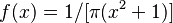# Cauchy distribution facts for kids

Kids Encyclopedia Facts

In mathematics, the Cauchy-Lorentz distribution (after Augustin-Louis Cauchy and Hendrik Lorentz) is a continuous probability distribution with two parameters: a location parameter and a scale parameter. As a probability distribution, it is usually called a Cauchy distribution. Physicists know it as a Lorentz distribution.

When the location parameter is 0 and the scale parameter is 1, the probability density function of the Cauchy distribution reduces to$f(x)= 1/[\pi (x^2+1)]$. This is called the standard Cauchy distribution.

The Cauchy distribution is used in spectroscopy to describe the spectral lines found there, and to describe resonance. It is also often used in statistics as the canonical example of a "pathological" distribution, since both its mean and its variance are undefined. The look of a Cauchy distribution is similar to that of a normal distribution, though with longer "tails".

## Images for kidsCauchy distribution Facts for Kids. Kiddle Encyclopedia.